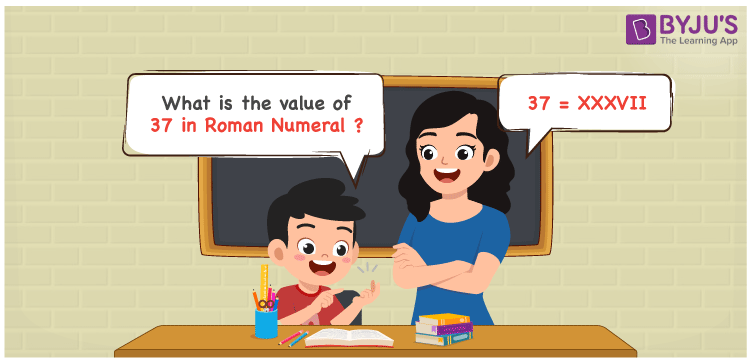# 37 in Roman Numerals

37 in Roman Numerals is XXXVII. To represent any number in Roman numerals, the 7 primary symbols are used with certain rules. Refer to the rules of Roman numerals. 37 is represented as XXXVII in the Roman numerals and it follows the addition rule. In the Roman numerals, XXXVII, 3 steps are followed. Step a) the numerals X is added thrice step b) The numeral V is added to twice I step 3) the resultant of step a) a and step b) are added. Lets understand it numerically in the next section.

 Number Roman Numeral 37 XXXVII

## How to Write 37 in Roman Numerals?To convert 37 in Roman Numerals, we need to split the number according to the available symbols. i,e I, V, X, L, C, D, and M.

The simple way to split 37 will be 30 + 7. (But there are no primary letters for 30 and 7).

Hence 37 can be represented as 10 + 10 + 10 + 5 + 1 + 1. So

37 = 10 + 10 + 10 + 5 + 1 + 1

37 = X + X + X + V + I + I

37 = XXXVII

## Video Lesson on Roman Numerals## Frequently Asked Questions on 37 in Roman Numerals

Q1

### How to write the number 37 in Roman Numerals?

37 in Roman Numerals is written as XXXVII.
Q2

### Does XXVI represent the number 37?

No. XXVI represents the number 26 and not 37.
Q3

### How to read the Roman numeral XXXVII in English?

The Roman numeral XXXVII should be read as Thirty Seven.